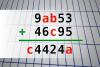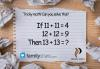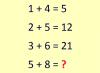BRAIN TEASERSBrain Teasers User Profile

# Chatchai Khumtaveeporn

rank
250
points
16
See full ranking list
short ranking list
 248 RAJAYOGAN P 16 249 adhi kurniawan 16 250 Chatchai Khumtaveeporn 16 251 VAHID KHALILI 16 252 Ognjen Cvetkovic 16
 Find number abc If 9ab53 + 46c95 = c4424a find number abc. Multiple solutions may exist.Can you solve this? Tricky math! Can you solve this? If 11+11=4 and 12+12=9 Then 13+13=?Calculate 5+8 If 1+4=5, 2+5=12 and 3+6=21 then 5+8=?